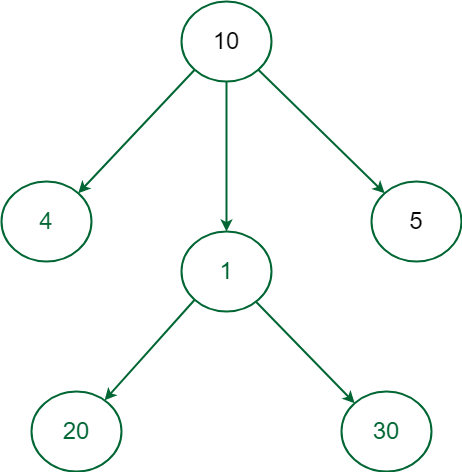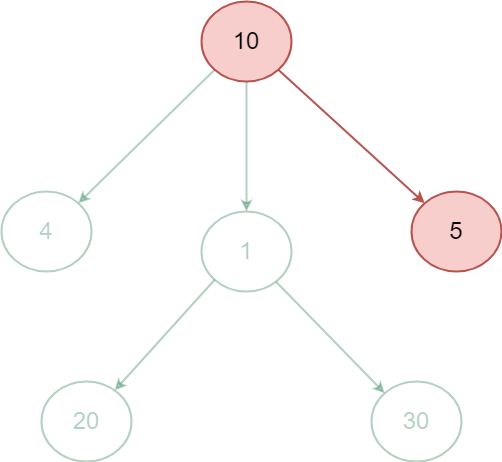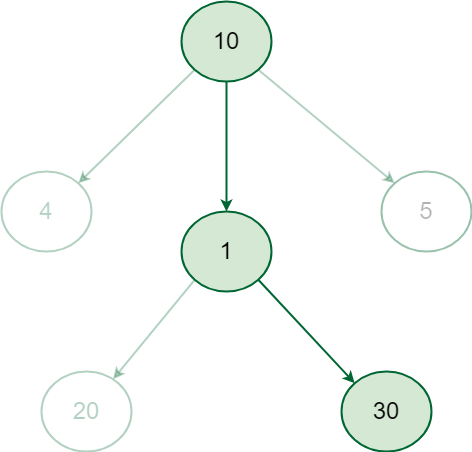GeeksforGeeks App
Open AppBrowser
Continue

# Introduction to Greedy Algorithm – Data Structures and Algorithm Tutorials

A greedy algorithm is an algorithmic paradigm that follows the problem-solving heuristic of making the locally optimal choice at each stage with the hope of finding a global optimum. In other words, a greedy algorithm chooses the best possible option at each step, without considering the consequences of that choice on future steps.

The greedy algorithm is useful when a problem can be divided into smaller subproblems, and the solution of each subproblem can be combined to solve the overall problem. The greedy algorithm can be used to solve optimization problems that involve finding the best solution among many possible solutions.

A classic example of a problem that can be solved using a greedy algorithm is the “coin change” problem. The problem is to make change for a given amount of money using the least possible number of coins. For example, if the amount is 25 cents and the available coins are 1 cent, 5 cents, and 10 cents, then the greedy algorithm would choose the largest coin possible at each step. It would start by selecting a 10-cent coin, then another 10-cent coin, and finally a 5-cent coin, for a total of three coins.

However, the greedy algorithm may not always find the optimal solution. For example, if the available coins are 1 cent, 3 cents, and 4 cents, and the amount is 6 cents, the greedy algorithm would select one 4-cent coin and two 1-cent coins , while the optimal solution is to use two 3-cent coins.

Therefore, it is important to prove the correctness of a greedy algorithm and to understand its limitations. The greedy algorithm can be applied in many contexts, including scheduling, graph theory, and dynamic programming.

Greedy Algorithm is defined as a method for solving optimization problems by taking decisions that result in the most evident and immediate benefit irrespective of the final outcome. It works for cases where minimization or maximization leads to the required solution.

## Characteristics of Greedy algorithm

For a problem to be solved using the Greedy approach, it must follow a few major characteristics:

• There is an ordered list of resources(profit, cost, value, etc.)
• Maximum of all the resources(max profit, max value, etc.) are taken.
• For example, in the fractional knapsack problem, the maximum value/weight is taken first according to available capacity.Introduction to Greedy Algorithm – Data Structures and Algorithm Tutorials

### Use of the greedy algorithm:-

The greedy algorithm is a method used in optimization problems where the goal is to make the locally optimal choice at each stage with the hope of finding a global optimum. It is called “greedy” because it tries to find the best solution by making the best choice at each step, without considering future steps or the consequences of the current decision.

Some common use cases for the greedy algorithm include:

Scheduling and Resource Allocation: The greedy algorithm can be used to schedule jobs or allocate resources in an efficient manner.

Minimum Spanning Trees: The greedy algorithm can be used to find the minimum spanning tree of a graph, which is the subgraph that connects all vertices with the minimum total edge weight.

Coin Change Problem: The greedy algorithm can be used to make change for a given amount with the minimum number of coins, by always choosing the coin with the highest value that is less than the remaining amount to be changed.

Huffman Coding: The greedy algorithm can be used to generate a prefix-free code for data compression, by constructing a binary tree in a way that the frequency of each character is taken into consideration.

It’s important to note that not all optimization problems can be solved by a greedy algorithm, and there are cases where the greedy approach can lead to suboptimal solutions. However, in many cases, the greedy algorithm provides a good approximation to the optimal solution and is a useful tool for solving optimization problems quickly and efficiently.

## All greedy algorithms follow a basic structure:

1. Declare an empty result = 0.
2. We make a greedy choice to select, If the choice is feasible add it to the final result.
3. return the result.

## Why choose Greedy Approach?

The greedy approach has a few tradeoffs, which may make it suitable for optimization. One prominent reason is to achieve the most feasible solution immediately. In the activity selection problem (Explained below), if more activities can be done before finishing the current activity, these activities can be performed within the same time.  Another reason is to divide a problem recursively based on a condition, with no need to combine all the solutions. In the activity selection problem, the “recursive division” step is achieved by scanning a list of items only once and considering certain activities.

## Greedy Algorithm Example:

Some Famous problems that exhibit Optimal substructure property and can be solved using Greedy approach are –

### 1) Job sequencing Problem:

Greedily choose the jobs with maximum profit first, by sorting the jobs in decreasing order of their profit. This would help to maximize the total profit as choosing the job with maximum profit for every time slot will eventually maximize the total profit

### 2) Prim’s algorithm to find Minimum Spanning Tree:

It starts with an empty spanning tree. The idea is to maintain two sets of vertices. The first set contains the vertices already included in the MST, the other set contains the vertices not yet included. At every step, it considers all the edges that connect the two sets and picks the minimum weight edge from these edges. After picking the edge, it moves the other endpoint of the edge to the set containing MST.

## How does the Greedy Algorithm works?

When the choice to apply the greedy method is made without conducting a thorough examination, the decision to utilize it can be somewhat difficult and occasionally even result in failure. In some cases taking the local best choice may lead to losing the global optimal solution.

For example:Graph with weighted vertices

• In the above graph starting from the root node 10 if we greedily select the next node to obtain the most weighted path the next selected node will be 5 that will take the total sum to 15 and the path will end as there is no child of 5 but the path 10 -> 5 is not the maximum weight path.Greedy Approach fails

• In order to find the most weighted path all possible path sum must be computed and their path sum must be compared to get the desired result, it is visible that the most weighted path in the above graph is 10 -> 1 -> 30 that gives the path sum 41Correct Approach

• In such cases Greedy approach wouldn’t work instead complete paths from root to leaf node has to be considered to get the correct answer i.e. the most weighted path, This can be achieved by recursively checking all the paths and calculating their weight.

Thus to use Greedy algorithm the problem must not contain overlapping subproblems.

## Greedy Algorithm Vs Dynamic Programming

Greedy algorithm and Dynamic programming are two of the most widely used algorithm paradigms for solving complex programming problems, While Greedy approach works for problems where local optimal choice leads to global optimal solution Dynamic Programming works for problems having overlapping subproblems structure where answer to a subproblem is needed for solving several other subproblems. Detailed differences are given in the table below:

## Greedy Algorithm Most Asked Interview Problems:

Some of the popular problems on the Greedy Approach that are widely asked in interviews are:

## Applications of Greedy Algorithms:

• Finding an optimal solution (Activity selection, Fractional Knapsack, Job Sequencing, Huffman Coding).
• Finding close to the optimal solution for NP-Hard problems like TSP.
• Greedy algorithm is used to select the jobs that will be completed before their respective deadlines and maximizes the profit.
• Greedy algorithms are used to cluster data points together based on certain criteria, such as distance or similarity.
•  The problem is broken down into smaller subproblems that are solved independently, but many of these subproblems are identical or overlapping.

## Advantages of the Greedy Approach:

• The greedy approach is easy to implement.
• Typically have less time complexity.
• Greedy algorithms can be used for optimization purposes or finding close to optimization in case of Hard problems.
• The greedy approach can be very efficient, as it does not require exploring all possible solutions to the problem.
• The greedy approach can provide a clear and easy-to-understand solution to a problem, as it follows a step-by-step process.
• The solutions to subproblems can be stored in a table, which can be reused for similar problems.

## Disadvantages of the Greedy Approach:

• The local optimal solution may not always be globally optimal.
• Lack of proof of optimality.
• The greedy approach is only applicable to problems that have the property of greedy-choice property meaning not all problems can be solved using this approach.
• The greedy approach is not easily adaptable to changing problem conditions.

### Here are some important points to keep in mind when working with greedy algorithms:

1. Greedy algorithms make the locally optimal choice at each step, without considering the consequences of that choice on future steps.
2. Greedy algorithms can be used to solve optimization problems that can be divided into smaller subproblems.
3. Greedy algorithms may not always find the optimal solution. It is important to prove the correctness of a greedy algorithm and to understand its limitations.
4. Greedy algorithms can be applied in many contexts, including scheduling, graph theory, and dynamic programming.
5. When designing a greedy algorithm, it is important to identify the optimal substructure and the greedy choice property.
6. The time complexity of a greedy algorithm depends on the specific problem and the implementation of the algorithm.
7. Greedy algorithms can sometimes be used as a heuristic approach to solve problems when the optimal solution is difficult to find in practice.
In some cases, a greedy algorithm may provide a solution that is close to the optimal solution, but not necessarily the exact optimal solution. These solutions are known as approximate solutions.

### Related Articles:

My Personal Notes arrow_drop_up